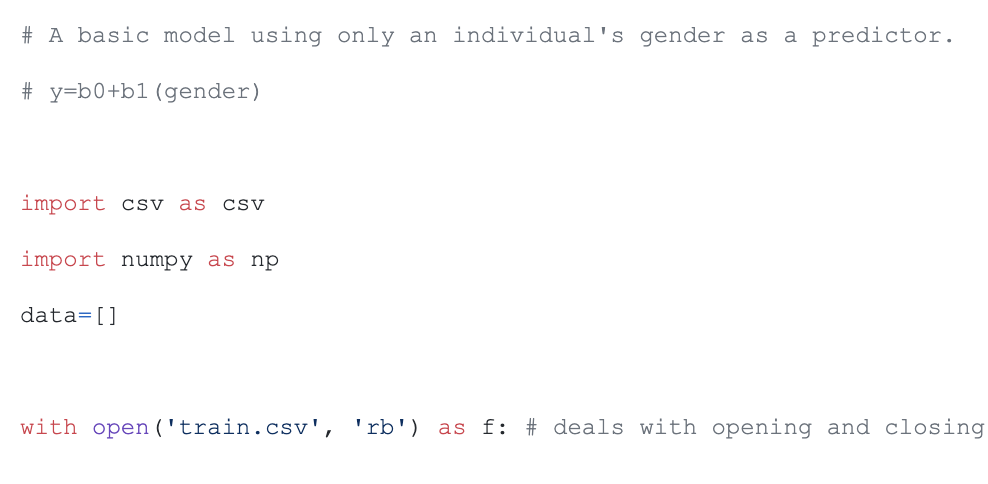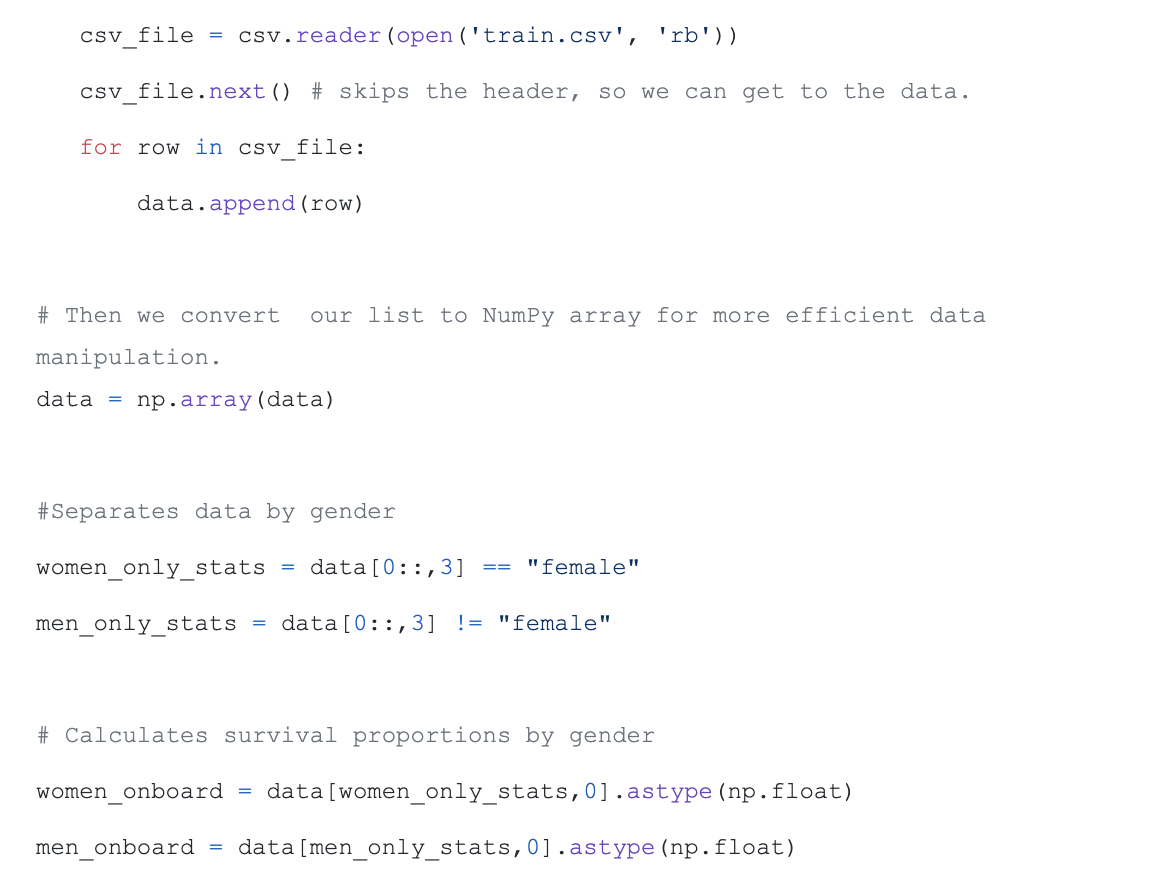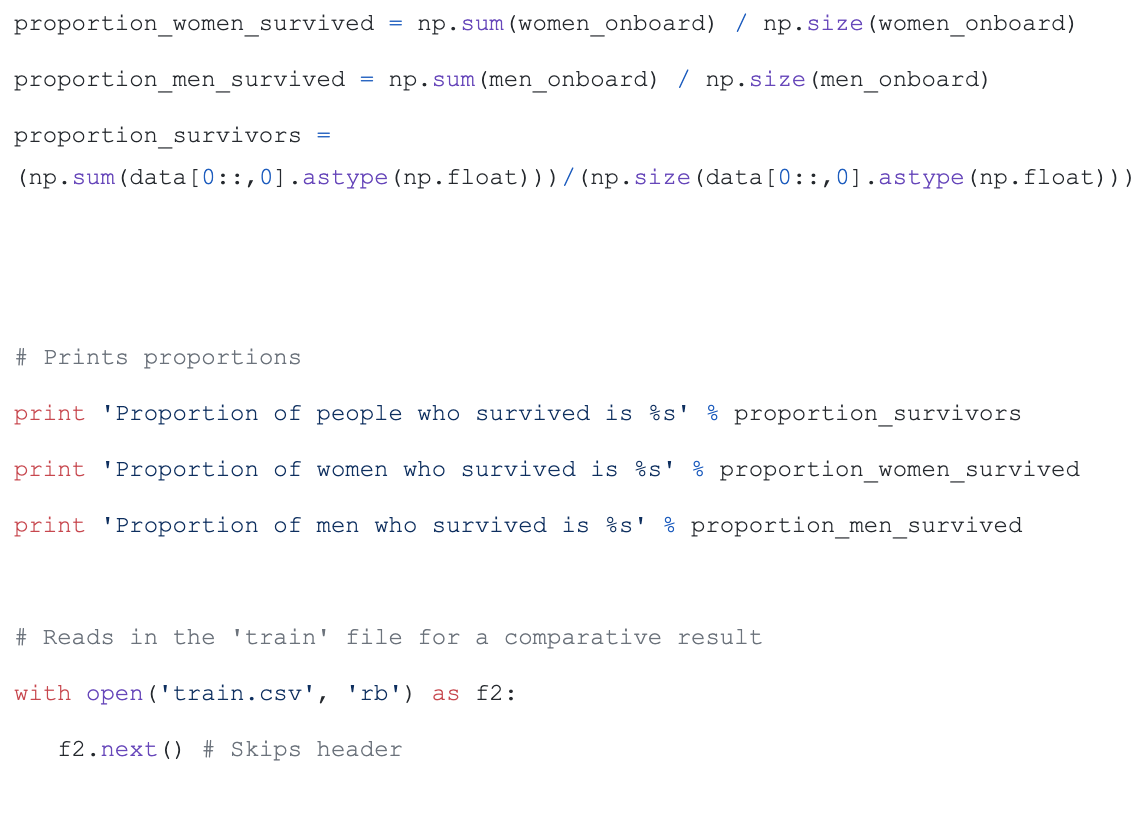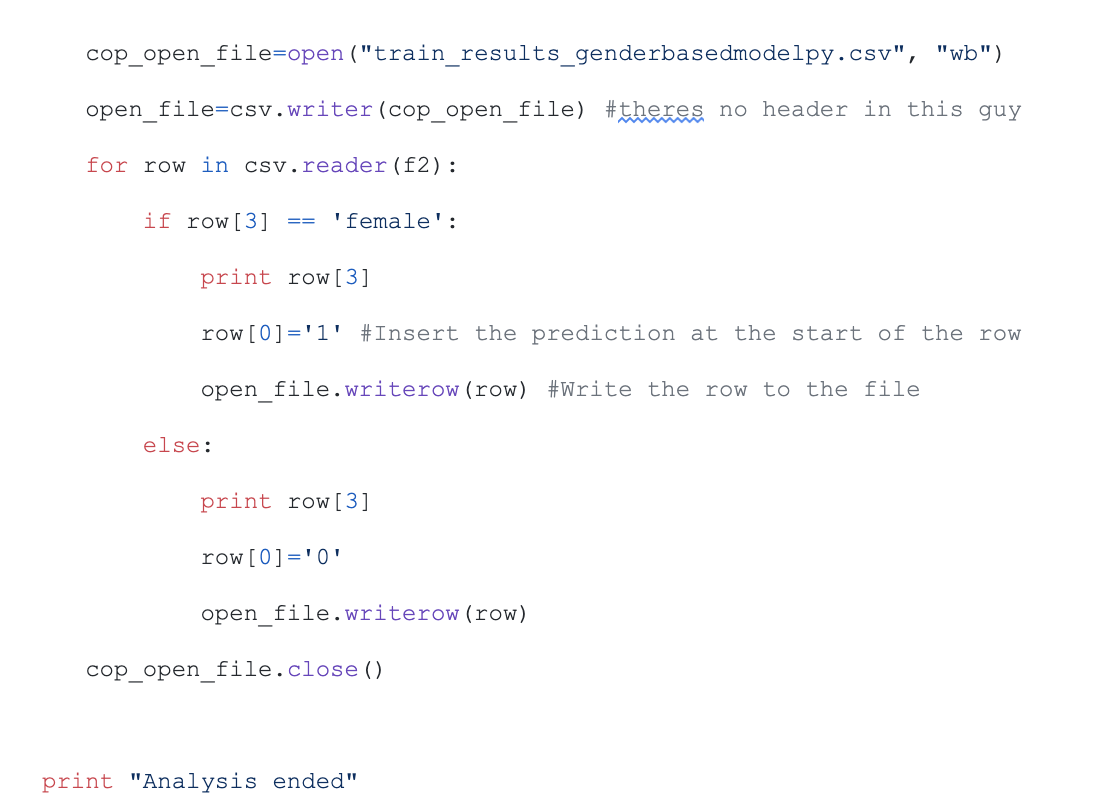# Working Under Supervision

## Introduction

Like it or not, most of us have a boss, and thus, we work under supervision. Our boss’s job is to make sure we stay focused and complete our work. We have quotas to fulfill and projects to complete. They know what the desired and expected outcomes are, the same way data scientists understand the result they are trying to produce with supervised learning.

## Supervised learning using Python

This blog is the third one of the series on learning Machine Learning using Python. In the first one, DataScience & Machine Learning: Where to start with Python, we covered setting up Python and installing the relevant libraries. In the second one Looking further into Machine Learning using Python, we covered different machine learning techniques and became familiar with supervised learning. We also talked about the scikit-learn toolkit and saw the SVM approach used due to its flexibility and usefulness.## Common Supervised Learning Techniques

In a broad sense, we will introduce some of the main techniques employed in supervised learning. To approximate an output based on the known answers, we can apply any of these supervised learning techniques. There are several more, but here we talk about some of the more common ones including:

• Linear regression
• Logistic regression
• Random forest
• K nearest neighbor
• Decision trees
• Support-vector machine
• Naive Bayes classifier

Tip: Regression methods are simple mathematical inductions to predict an output based on input. These techniques work when you know the input and are trying to estimate the output.

#### Linear Regression

Linear regression is the ability to predict a value based on a straight line drawn between the X-axis and Y-axis with a set of known values. For instance, the typical example used is how to identify a house’s sale value based on the square feet of built-up area. Machine learning, however, tries to tackle more complex questions, questions that may take much more time if a human were doing them by hand.

#### Logistic Regression

Now that we have explained linear regression let us talk about logistic regression. Here we draw a curve using a superimposed mathematical equation to join together the known values to guess an unknown. There are also many other methods called ridge linear regression, lasso regression, and others that functionally will map together points and allow for an estimated output.

#### Random Forest

Another often used algorithm is called the Random forest. Here we aggregate all values into groups based on a Euclidean distance concept and try to minimize the error based on feedback.

Tip: Supervised learning methods, such as the ones here, have the benefit of estimating error, unlike with unsupervised learning. So you will be able to know how close to the correct output you are.

#### K-nearest Neighbor

KNN or K-nearest neighbor algorithm is the idea of refining the random forest algorithm seen above by grouping together the neighboring data values. In my research, it appears a lot more widely used than all the other methods discussed above. KNN reduces the dimensionality of the input data and uses proximity as a strategy to obtain training. The quality of the training varies from problem to problem, and we must continually evaluate our results against tests of the real data to choose the strategy that gives us the closest results.

#### Decision Trees

Decision trees are similar to the k-nearest neighbors in their ability to go to multiple branches based on the flowchart approach. They are simple yet handy.

#### SVM Support Vector Method

When we talked about the Scikit-learn toolkit in the previous blog, we mostly saw the SVM support vector method approach used due to its flexibility and usefulness. SVM allows us to apply a model, then transform and fit to get values. We also often reduce the dimensions and varying values over a long-range and shrink them to focus on only specific columns in a big matrix of values.

#### Naive Bayes

Naive Bayes is a probability theorem often used for classification problems. Most often, it appears in spam detection and text classification.

Other machine learning topics we didn’t cover today are neural networks and deep learning, which are a more advanced form of supervised learning. Shaswat, one of our data engineers, has covered these topics in his blog: Artificial Intelligence & Everyday Life!

Though this was a short introduction to these machine learning techniques, I want to be clear that they are compelling and allow for many machine learning algorithms to work today successfully.

## Illustrating the Power of Supervised LearningThis source code is from the GitHub project kaggle-titanic. This is real data from the Titanic shipwreck.

The code uses machine learning to predict the probability of a specific gender surviving a situation. Here there are only two possibilities: either more men survive or more women survive. The advantage we have is that we know the answers already due to the training and test data. We, thus, can quickly and quite accurately figure out if we are using the correct model or not.

This is the genius of supervision; we have a reliable feedback mechanism to judge our inner workings of the algorithm. We know what the desired outcome is and thus can decide very quickly how accurate we are. Unsupervised learning does not include feedback to evaluate its effectiveness and, therefore, it only works when the assumptions used are valid for each situation.

## Summary

In this blog, we learned about the most used supervised learning algorithms and got an opportunity to write Python code to try and test them.

In a way, supervised learning is like attending an open book exam. But remember, even if it is a brilliant method to classify or categorize input data, we still can’t know for sure how well it will perform under new unseen data conditions.